Worksheet Solution: Addition (Double Digit)

# Worksheet Solution: Addition (Double Digit) Notes | Study Mathematics for Class 1: NCERT - Class 1

## Document Description: Worksheet Solution: Addition (Double Digit) for Class 1 2022 is part of Mathematics for Class 1: NCERT preparation. The notes and questions for Worksheet Solution: Addition (Double Digit) have been prepared according to the Class 1 exam syllabus. Information about Worksheet Solution: Addition (Double Digit) covers topics like and Worksheet Solution: Addition (Double Digit) Example, for Class 1 2022 Exam. Find important definitions, questions, notes, meanings, examples, exercises and tests below for Worksheet Solution: Addition (Double Digit).

Introduction of Worksheet Solution: Addition (Double Digit) in English is available as part of our Mathematics for Class 1: NCERT for Class 1 & Worksheet Solution: Addition (Double Digit) in Hindi for Mathematics for Class 1: NCERT course. Download more important topics related with notes, lectures and mock test series for Class 1 Exam by signing up for free. Class 1: Worksheet Solution: Addition (Double Digit) Notes | Study Mathematics for Class 1: NCERT - Class 1
 1 Crore+ students have signed up on EduRev. Have you?

Q.1. Find the sum: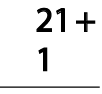Ans.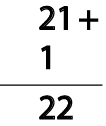Q.2. Find the sum: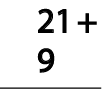Ans.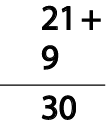Q.3. Find the sum: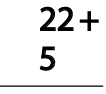Ans.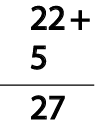Q.4. Find the sum: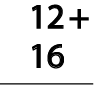Ans.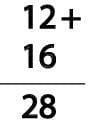Q.5. Find the sum: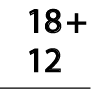Ans.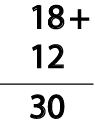Q.6. Find the sum: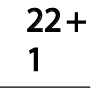Ans.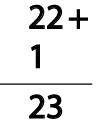Q.7. Find the sum: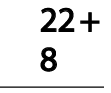Ans.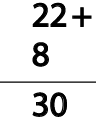Q.8. Find the sum: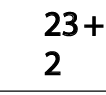Ans.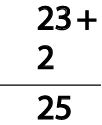Q.9. Find the sum: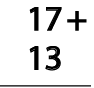Ans.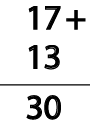Q.10. Find the sum: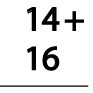Ans.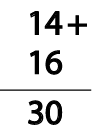The document Worksheet Solution: Addition (Double Digit) Notes | Study Mathematics for Class 1: NCERT - Class 1 is a part of the Class 1 Course Mathematics for Class 1: NCERT.
All you need of Class 1 at this link: Class 1

## Mathematics for Class 1: NCERT

47 videos|59 docs|25 tests
 Use Code STAYHOME200 and get INR 200 additional OFF

## Mathematics for Class 1: NCERT

47 videos|59 docs|25 tests

Track your progress, build streaks, highlight & save important lessons and more!

,

,

,

,

,

,

,

,

,

,

,

,

,

,

,

,

,

,

,

,

,

;